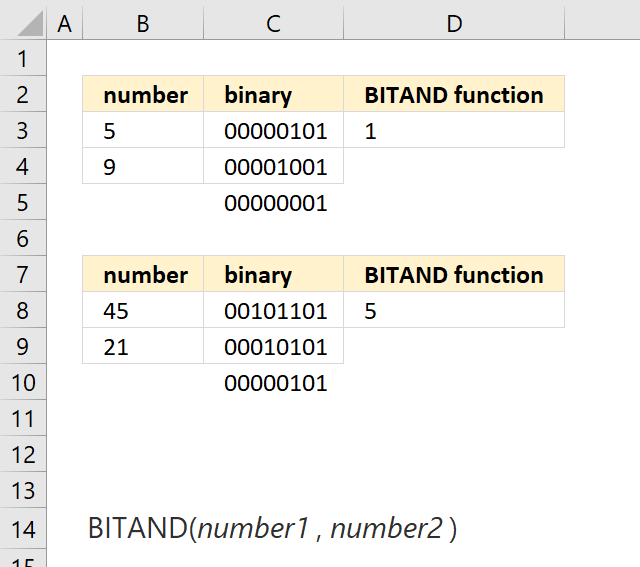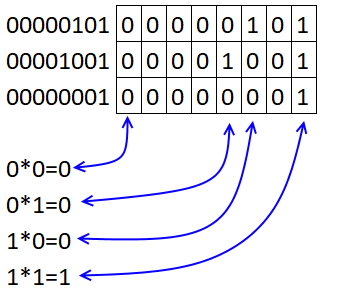Author: Oscar Cronquist Article last updated on May 11, 2022The BITAND function calculates a bitwise 'AND' of two numbers.

The function performs AND logic bit by bit on the numbers based on their binary representation. AND logic means that the value of each bit position is counted only if both parameter's bits at that position are 1.

Formula in cell B3:

=BITAND(B3, B4)

Number 5 is 00000101 and 9 is 00001001. With AND logic bitwise the result is 00000001 which is number 1.### Excel Function Syntax

BITAND(number1number2)

### Arguments

 number1 Required. The first number. number2 Required. The second number.